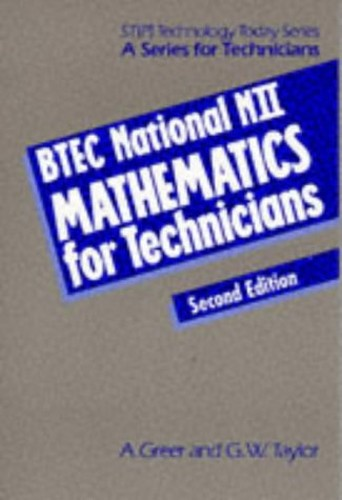# BTEC National NII Mathematics for Technicians by Alan Fuller

In Stock
\$10.99
+10% GST
This textbook contains everything students need for the core Mathematics units covered in any BTEC National Engineering course. It combines material from BTEC National NII and NIII Mathematics for Technicians to provide one complete volume for Level 3 Engineering.
11 in stock

## BTEC National NII Mathematics for Technicians Summary

### BTEC National NII Mathematics for Technicians by Alan Fuller

Completely up-to-date with the latest Edexcel BTEC specifications. Covers basic mathematic operations including indices, transposition of formulae, scientific units, logarithms and exponents. Contains a new chapter on the use of a scientific calculator. Presents this sometimes difficult subject in a simple and user-friendly way with over 300 diagrams to explain key topics.

### Why buy from World of BooksOur excellent value books literally don't cost the earthFree delivery in AustraliaEvery used book bought is one saved from landfill

Calculation - approximation and accuracy, the scientific calculator, units, tables and charts; logic design and number systems; algebra - indices (revision), basic operations (revision), solving linear equations (revision), transposition of formulae, quadratic equations, logarithms and exponentials; trigonometry - radian measure, triangles and waveforms, practical applications of trigonometry, three-dimensional (solid) trigonometry; mensuration - areas and volumes, volumes and surface areas (Pappus theorems); graphs - graphs of linear equations, graphs of non-linear equations, graphical solution of equations, polar graphs; statistics - data collection and analysis; calculus - differentiation, application of calculus to problems, integration; vectors; matrices and determinants; complex numbers.

GOR001214004
BTEC National NII Mathematics for Technicians by Alan Fuller
Alan Fuller
Used - Very Good
Paperback
Oxford University Press
1994-04-01
456
0748717013
9780748717019
N/A
Book picture is for illustrative purposes only, actual binding, cover or edition may vary.
This is a used book - there is no escaping the fact it has been read by someone else and it will show signs of wear and previous use. Overall we expect it to be in very good condition, but if you are not entirely satisfied please get in touch with us.